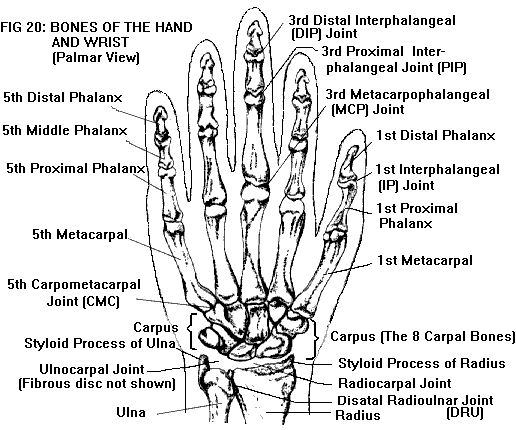# Five digits in our right handLet $x$ denote the sum of all digits of $7777^{7777}$. Now, let $y$ be the sum of all digits of $x$. Similarly, let $z$ denote the sum of all digits of $y$. What is the sum of all digits of $z$?

×